# Go Math Grade 3 Chapter 6 Answer Key Pdf Understand Division

## Understand Division Go Math Grade 3 Chapter 6 Answer Key Pdf

Check out the topics covered in the Grade 3 Go Math Answer Key Chapter Understand Division from the below section. Parents can guide your child by making our Go Math Grade 3 Chapter 6 Key as reference. Students who are willing to score good marks in exams are suggested to practice the problems given in our Go Math Answer Key. Access the links given below and Download Go Math 3rd Grade Chapter 6 Solution Key pdf.

Lesson 1: Problem Solving • Model Division

Lesson 2: Size of Equal Groups

Lesson 3: Number of Equal Groups

Lesson 4: Model with Bar Models

Lesson 5: Algebra • Relate Subtraction and Division

Mid-Chapter Checkpoint

Lesson 6: Investigate • Model with Arrays

Lesson 7: Algebra • Relate Multiplication and Division

Lesson 8: Algebra • Write Related Facts

Lesson 9: Algebra • Division Rules for 1 and 0

Chapter 6 Review/Test

### Model Division Page No 305

Question 1.
Six customers at a toy store bought 18 jump ropes. Each customer bought the same number of jump ropes. How many jump ropes did each customer buy?
__________

Explanation:

Given that there are Six customers at a toy store bought 18 jump ropes
Each customer bought the same number of jump ropes
To know the number of jump roses that each customer bought
You must place each jump ropes until all the jump ropes are used.
That means 18 jump ropes to all 6 customers
= 18 ÷ 6 = 3
Therefore each customer bought 3 jump ropes

Question 2.
Hiro has 36 pictures of his summer trip. He wants to put them in an album. Each page of the album holds 4 pictures. How many pages will Hiro need for his pictures?
__________

Explanation:

Hiro has 36 pictures of his summer trip. He wants to put them in an album
Each page of the album holds 4 pictures
Make it into the equal groups and put 4 pictures in each page
= 36 ÷ 4 = 9
Thus Hiro need 9 pages for his pictures

Question 3.
Katia has 42 crayons in a box. She buys a storage bin that has 6 sections. She puts the same number of crayons in each section. How many crayons does Katia put in each section of the storage bin?
__________

Explanation:

Katia has 42 crayons in a box
She buys a storage bin that has 6 sections
Make 42 crayons as a group and place 1 crayon in each section. Then you get 7 crayons in each section
42 ÷ 6 = 42/6 = 7
Therefore 7 crayons do Katia put in each section of the storage bin

Go Math Grade 3 Chapter 6 Understand Division Question 4.
Ms. Taylor’s students give cards to each of the 3 class parent helpers. There are 24 cards. How many cards will each helper get if the students give an equal number of cards to each helper?
__________

Explanation:

Ms. Taylor’s students give cards to each of the 3 class parent helpers
Total number of cards = 24
Make 3 equal groups and place each card for 3 class parent helpers
You get 8 cards for each helper
24 ÷ 3 = 8

Question 5.
Jamie divides 20 baseball stickers equally among 5 of his friends. How many stickers does each friend get?
__________

Explanation:

Jamie divides 20 baseball stickers equally among 5 of his friends
Give each baseball sticker to each friend and repeat the process until you finish all the stickers
So, each friend gets 4 baseball stickers.
20 ÷ 5 = 4 stickers

### Model Division Page No 306

Question 1.
Maria buys 15 apples at the store and places them into bags. She puts 5 apples into each bag. How many bags does Maria use for all the apples?
Options:
a. 2
b. 3
c. 4
d. 10

Explanation:

Maria buys 15 apples at the store and places them into bags
She puts 5 apples into each bag
That means each bag contains 5 apples
Now, Divide the apples into equal groups and place them in each bag
15 ÷ 5 = 3
Therefore Maria uses 3 bags to put 15 apples.

Question 2.
Tom’s neighbor is fixing a section of his walkway. He has 32 bricks that he is placing in 8 equal rows. How many bricks will Tom’s neighbor place in each row?
Options:
a. 3
b. 4
c. 5
d. 6

Explanation:

Given: Tom’s neighbor is fixing a section of his walkway
He has 32 bricks that he is placing in 8 equal rows
Now place each brick in all 8 rows  equally and repeat until the bricks are over
32 ÷ 8 = 4
So, you get 4 bricks in each row
Thus the correct answer is option B

Question 3.
Find the unknown factor.
7 × _ = 56
Options:
a. 6
b. 7
c. 8
d. 9

Explanation:

Let the unknown factor be x
7 × x = 56So, the correct answer is option C

Question 4.
How many students practiced the piano more than 3 hours a week?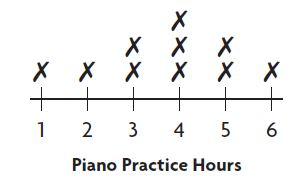Options:
a. 2
b. 6
c. 8
d. 10

Explanation:

3 students practiced the piano for 4 hours
2 students practiced the piano for 5 hours and
1 student practiced the piano for 6 hours
= 3 + 2 + 1 = 6
The above line plot shows that there are 6 students who practiced the piano for more than 3 hours

Count equal groups to find how many there are.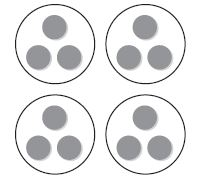Options:
a. 3
b. 4
c. 12
d. 16

Explanation:

The above figure shows that there are 4 circles and each circle is divided into 3 equal groups
So, multiply the number of circles by equal groups
= 4 × 3 = 12
Thus the correct answer is option C

Question 6.
Which is another way to group the factors?
(3 × 2) × 5
Options:
a. (3 + 2) + 5
b. (3 × 2) + 5
c. 3 × (2 + 5)
d. 3 × (2 × 5)

Answer: 3 × (2 × 5)

Explanation:

The another way to group (3 × 2) × 5 is 3 × (2 × 5)
Because there is no change in the symbol and number
So, the correct answer is option D

### Size of Equal Groups Page No 311

Use counters or draw a quick picture. Make equal groups. Complete the table.

Question 1.

 Counters Number of Equal Groups Number in Each Group 1. 15 3 __________ 2. 21 7 __________ 3. 28 7 __________ 4. 32 4 __________ 5. 9 3 __________ 6. 18 3 __________ 7. 20 5 __________ 8. 16 8 __________ 9. 35 5 __________ 10. 24 3 __________

 Counters Number of Equal Groups Number in Each Group 1. 15 3 5 2. 21 7 3 3. 28 7 4 4. 32 4 8 5. 9 3 3 6. 18 3 6 7. 20 5 4 8. 16 8 2 9. 35 5 7 10. 24 3 8

Explanation:

1. No. of counters = 15
Number of equal groups = 3
Place 1 counter to each group, you get 5 in each group

2. No. of counters = 21
Number of equal groups = 7
Place 1 counter to each group, you get 3 in each group

3. No. of counters = 28
Number of equal groups = 7
Place 1 counter to each group, you get 4 in each group

4. No. of counters = 32
Number of equal groups = 4
Place 1 counter to each group, you get 8 in each group

5. No. of counters = 9
Number of equal groups = 3
Place 1 counter in each group, you get 3 in each group

6. No. of counters = 18
Number of equal groups = 3
Place 1 counter to each group, you get 6 in each group

7. No. of counters = 20
Number of equal groups = 5
Place 1 counter to each group, you get 4 in each group

8. No. of counters = 16
Number of equal groups = 8
Place 1 counter to each group, you get 2 in each group

9. No. of counters = 35
Number of equal groups = 5
Place 1 counter to each group, you get 7 in each group

10. No. of counters = 24
Number of equal groups = 3
Place 1 counter to each group, you get 8 in each group

Question 11.
Alicia has 12 eggs that she will use to make 4 different cookie recipes. If each recipe calls for the same number of eggs, how many eggs will she use in each recipe?
__________

Explanation:

The total number of eggs Alicia has = 12
she will use it to make 4 different cookie recipes
Make 4 groups and place 12 eggs in each group
You get 3 eggs in each group
12/4 = 3 eggs

Go Math Grade 3 Chapter 6 Pdf Question 12.
Brett picked 27 flowers from the garden. He plans to give an equal number of flowers to each of the 3 people. How many flowers will each person get?
__________

Explanation:

Given,
Brett picked 27 flowers from the garden
He plans to give an equal number of flowers to each of 3 people
Give each flower to 3 people until 27 flowers complete
So, Each person get 9 flowers
27 ÷ 3 = 9 flowers

### Size of Equal Groups Page No 312

Question 1.
Ryan has 21 pencils. He wants to put the same number of pencils in each of the 3 pencil holders. How many pencils will he put in each pencil holder?
Options:
a. 6
b. 7
c. 8
d. 9

Explanation:

Ryan has 21 pencils
He wants to put the same number of pencils in each of 3 pencil holders
Split it into 3 groups and share each pencil with each group
You get 7 pencils for each of 3 groups
21/3 = 7
Thus the correct answer is option B

Question 2.
Corrine is setting out 24 plates on 6 tables for dinner. She sets the same number of plates on each table. How many plates does Corrine set on each table?
Options:
a. 3
b. 4
c. 5
d. 6

Explanation:

Given,
Corrine is setting out 24 plates on 6 tables for a dinner
She sets the same number of plates on each table
Make a group of 6 tables and place 1 plate for each group
You get 4 plates for each table
24/6 = 4 plates
So, the correct answer is Option B

Question 3.
Each table has 4 legs. How many legs do 4 tables have?
Options:
a. 1
b. 8
c. 16
d. 20

Explanation:

Given that Each table has 4 legs
4 tables have x number of legs
x = 4 × 4 = 16
Therefore 4 tables have 16 legs

Question 4.
Tina has 3 stacks of 5 CDs on each of 3 shelves. How many CDs does she have in all?
Options:
a. 14
b. 30
c. 35
d. 45

Given,
Tina has 3 stacks of 5 CDs on each of 3 shelves
How many CDs does she have in all = x
To know the number of CDs in all we have to multiply no. of stacks, no. of CDs on each of 3 shelves
x = 3 × 5 × 3 = 15 × 3 = 45
So, the answer is option D

Question 5.
What is the unknown factor?
7 × _= 35
Options:
a. 4
b. 5
c. 6
d. 7

Explanation:

Let the unknown factor be x
7 × x = 35
x = 35/7 = 5
Therefore the correct answer is option B

Question 6.
Which of the following describes a pattern in the table?

 Number of packs 1 2 3 4 5 Number of yo-yos 3 6 9 12 ?

Options:
b. Multiply by 2.
c. Multiply by 3.

Explanation:

Add 3 yo-yos for each pack and multiply no. of pans by 3

### Number of Equal Groups Page No 317

Draw counters on your MathBoard. Then circle equal groups. Complete the table.

Question 1.

 Counters Number of Equal Groups Number in Each Group 1. 24 3 8 2. 35 __________ 7 3. 30 __________ 5 4. 16 __________ 4 5. 12 __________ 6 6. 36 __________ 9 7. 18 __________ 3 8. 15 __________ 5 9. 28 __________ 4 10. 27 __________ 3

 Counters Number of Equal Groups Number in Each Group 1. 24 3 8 2. 35 5 7 3. 30 6 5 4. 16 4 4 5. 12 2 6 6. 36 4 9 7. 18 6 3 8. 15 3 5 9. 28 7 4 10. 27 9 3

Explanation:

1. No. of counters = 24
Number in each group = 8
24/8 = 3
So, the number of equal groups = 3

2. No. of counters = 35
Number in each group = 7
35/7 = 5
So, the number of equal groups = 5

3. No. of counters = 30
Number in each group = 5
30/5 = 6
So, the number of equal groups = 6

4. No. of counters = 16
Number in each group = 4
16/4 = 4
So, the number of equal groups = 4

5. No. of counters = 12
Number in each group = 6
12/6 = 2
So, the number of equal groups = 2

6. No. of counters = 36
Number in each group = 9
36/9 = 4
So, the number of equal groups = 4

7. No. of counters = 18
Number in each group = 3
18/3 = 6
So, the number of equal groups = 6

8. No. of counters = 15
Number in each group = 5
15/5 = 3
So, the number of equal groups = 3

9. No. of counters = 28
Number in each group = 4
28/4 = 7
So, the number of equal groups = 7

10. No. of counters = 27
Number in each group = 3
27/3 = 9
So, the number of equal groups = 9

In his bookstore, Toby places 21 books on shelves, with 7 books on each shelf. How many shelves does Toby need?
__________

Explanation:

Toby places 21 books on shelves, with 7 books on each shelf
No. of shelves does Toby need = x
x = 21/7 = 3
Thus Toby needs 3 shelves

Question 12.
Mr. Holden has 32 quarters in stacks of 4 on his desk. How many stacks of quarters are on his desk?
__________

Explanation:

Mr. Holden has 32 quarters in stacks of 4 on his desk
No. of stacks of quarters on his desk = y
y × 4 = 32
y = 32/4 = 8 stacks
No. of stacks of quarters on his desk = 8 stacks

### Number of Equal Groups Page No 318

Question 1.
Ramon works at a clothing store. He puts 24 pairs of jeans into stacks of 8. How many stacks does Ramon make?
Options:
a. 5
b. 4
c. 3
d. 2

Explanation:

Ramon puts 24 pairs of jeans into stacks of 8
Split the pair of jeans to 8 groups of the stack
You get 3 pair of jeans for each stack
24/8 = 3
Therefore the correct answer is option C

Question 2.
There are 36 people waiting in line for a hayride. Only 6 people can ride on each wagon. If each wagon is full, how many wagons are needed for all 36 people?
Options:
a. 5
b. 6
c. 7
d. 8

Explanation:

There are 36 people waiting in line for a hayride.
Only 6 people can ride on each wagon
Split 36 people into 6 groups
That means 36/6 = 6 Wagons
So, the correct answer is option B

Question 3.
Which multiplication sentence does the array show?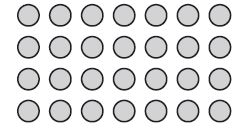Options:
a. 4 × 5 = 20
b. 4 × 6 = 24
c. 4 × 7 = 28
d. 4 × 8 = 32

Answer: 4 × 7 = 28

Explanation:

There are 4 rows and 7 columns
rows × columns = 4 × 7 = 28
Thus the answer is option C

Question 4.
Austin buys 4 boxes of nails for his project. There are 30 nails in each box. How many nails does Austin buy in all?
Options:
a. 12
b. 34
c. 70
d. 120

Explanation:

Given,
Austin buys 4 boxes of nails for his project
There are 30 nails in each box
No. of nails does Austin buy in all = 30 + 30 + 30 + 30
4 × 30 = 120
So, the correct answer is option D

Question 5.
Which describes the number sentence?
8 + 0 + 8
Options:
a. odd + odd = odd
c. even + even = even

Explanation:

In math, identity is a number, n, that when added to other numbers, gives the same number, n. The additive identity is always zero. This brings us to the identity property of addition, which simply states that when you add zero to any number, it equals the number itself.

Question 6.
Each month for 6 months, Kelsey completes 5 paintings. How many more paintings does she need to complete before she has completed 38 paintings?
Options:
a. 2
b. 6
c. 8
d. 9

Explanation:

Each month for 6 months
Kelsey completes 5 paintings
x no. of paintings she needs to complete before she has completed 38 paintings
6 × 5 = 30 paintings
x + 30 = 38
x = 38 – 30
x = 8
So, the correct answer is option C

### Model with Bar Models Page No 323

Write a division equation for the picture.

Question 1.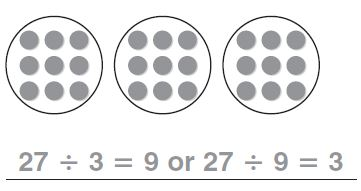Answer: 27 ÷ 3 = 9 or 27 ÷ 9 = 3

Explanation:

There are 27 counters and each circle has 9 groups. There are 3 groups of 9 counters.
27 ÷ 3 = 27/3 = 9
27 ÷ 9 = 27/9 = 3

Question 2.Type below:
___________

Answer: 16 ÷ 8 = 2 or 16 ÷ 2 = 8

Explanation:

The total number of counters is 16 and circle a group of 2 counters. Continue circling groups of 2 until all 16 counters are in the group.
So, Divide Total number of counters by number of equal groups
16 ÷ 8 = 16/8 = 2
Next, Divide total number of counters by no. of counters in each group.
16 ÷ 2 = 16/2 = 8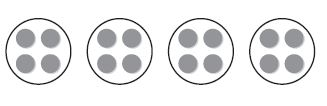Type below:
___________

Answer: 16 ÷ 4 = 4

Explanation:

The total number of counters is 16 and circle a group of 4 counters. Continue circling groups of 4 until all 16 counters are in the group
Divide No. of counters by no. of equal groups = 16 ÷ 4 = 4
And then divide no. of counters by no. of counters in each group = 16 ÷ 4 = 4

Question 4.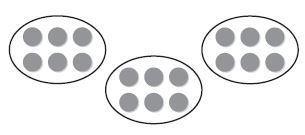Type below:
___________

Answer: 18 ÷ 3 = 6 or 18 ÷ 6 = 3

Explanation:

Number of counters = 18
Number of Equal groups = 3
Number of counters in each group = 6
Divide No. of counters by no. of equal groups
18 ÷ 3 = 18/3 = 6
Divide No. of counters by no. of counters in each group
18 ÷ 6 = 18/6 = 3

Complete the bar model to solve. Then write a division equation for the bar model.

Question 5.
There are 15 postcards in 3 equal stacks. How many postcards are in each stack?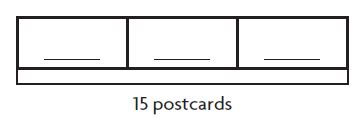________ postcards

Answer: 5 postcards, 15 ÷ 3 = 5

Explanation:

Total number of postcards = 15
Number of equal stacks = 3
Number of postcards in each stack = x
x = No. of postcards/ No. of equal stacks
x = 15/3
x = 5
Thus each stack contains 5 postcards

Question 6.
There are 21 key rings. How many groups of 3 key rings can you make?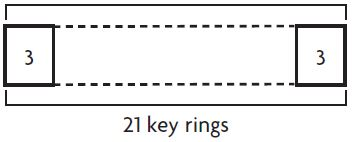________ groups

Answer: 7 groups, 21 ÷ 3 = 7

Explanation:

Divide the 21 key rings into 7 to make 7 groups with 3 key rings each group
21 ÷ 3 = 21/3 = 7 groups

There are 7 groups of 3 key rings.

Problem Solving

Question 7.
Jalyn collected 24 stones. She put them in 4 equal piles. How many stones are in each pile?
________ stones

Explanation:

Given,

Jalyn collected 24 stones
She put them in 4 equal piles
No. of stones in each pile = x
Divide total no. of stones by 4 equal piles
24 ÷ 4 = 24/4 = 6
There are 6 stones in each pile

Question 8.
Tanner has 30 stickers. He puts 6 stickers on each page. On how many pages does he put stickers?
________ pages

Explanation:

Tanner has 30 stickers
Tanner puts 6 stickers on each page
Now, Divide Number of stickers by number of stickers on each page
= 30 ÷ 6 = 30/6 = 5 pages

### Model with Bar Models Page No 324

Question 1.
Jack and his little sister are stacking 24 blocks. They put the blocks in 3 equal stacks. How many blocks are in each stack?
Options:
a. 4
b. 6
c. 7
d. 8

Explanation:

Total number of blocks = 24
They put the blocks in 3 equal stacks
To know the blocks in each stack, we have to divide no. of blocks by number of equal stacks
24 ÷ 3 = 24/3 = 8 blocks
There are 8 blocks in each stack
So, the correct answer is option D

Question 2.
Melissa made 45 greeting cards. She put them in 5 equal piles. How many cards did she put in each pile?
Options:
a. 9
b. 8
c. 7
d. 6

Explanation:

Given,
She put them in 5 equal piles
To know the number of cards she put in each pile
We have to divide number of cards by no. of equal piles
= 45 ÷ 5 = 45/5 = 9 cards
Thus there are 9 cards in each pile
So, the correct answer is option A

Spiral Review

Question 3.
Angie puts 1 stamp on each envelope. She puts stamps on 7 envelopes. How many stamps does Angie use?
Options:
a. 0
b. 1
c. 7
d. 8

Explanation:

Angie puts 1 stamp on each envelope
She puts stamps on 7 envelopes
To find Number of stamps Angie use, we have to divide no. of stamps on envelopes by no. of stamps on each envelope
= 7 ÷ 1 = 7

Question 4.
A carnival ride has 8 cars. Each car holds 4 people. How many people are on the ride if all the cars are full?
Options:
a. 34
b. 32
c. 28
d. 24

Explanation:

Given,
A carnival ride has 8 cars
Each car holds 4 people
1 car = 4 people
8 cars = x
x × 1 = 4 × 8
x = 32
32 people are on the ride if all the cars are full

Use the line plot for 5–6.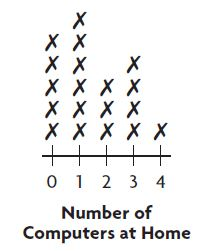Question 5.
How many families have 1 computer at home?
Options:
a. 4
b. 5
c. 6
d. 7

Explanation:

The line plot shows that there are 6 families who have 1 computer at home

Question 6.
How many families have more than 1 computer at home?
Options:
a. 4
b. 5
c. 7
d. 8

Explanation:

Number of Families have 2 computers at home = 3
Number of Families have 3 computers at home = 4
Number of Families have 4 computers at home = 1
Number of Families have more than 1 computer at home = 3 + 4 + 1 = 8

### Relate Subtraction and Division Page No 329

Write a division equation.

Question 1.Answer: 16 ÷ 4 = 4

Explanation:

Step 1:

Step 2:

Subtract with 4 until you get 0

Step 3:

Count the number of times you subtract 4

Since you subtract 4 times
There are 4 groups 4 in 16
So 16 ÷ 4 = 4
Sixteen divided by four equals four

Question 2.______ ÷ ______ = ______

Answer: 12 ÷ 3 = 4

Explanation:

Step 1:

Start at 12

Step 2:

Count back by 3s as many times as you can.

Step 3:

Count the number of times you jumped back 3.

You jumped back 3 four times
There are 4 groups of 3 in 12
12 ÷ 3 = 4______ ÷ ______ = ______

Answer: 10 ÷ 2 = 5

Explanation:

Step 1:

Start at 10

Step 2:

Count back by 2s as many times as you can.

Step 3:

Count the number of times you jumped back 2.

You jumped back 2 five times
There are 5 groups of 2 in 10
10 ÷ 2 = 5

Question 4.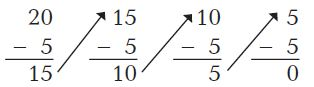______ ÷ ______ = ______

Answer: 20 ÷ 5 = 4

Explanation:

Step 1:

Start at 20

Step 2:

Subtract with 5 until you get 0

Step 3:

Count the number of times you subtract with 5

Since you subtract 4 times
There are 4 groups of 5 in 20
So 20 ÷ 5 = 4
Twenty divided by five equals four

Use repeated subtraction or a number line to solve.

Question 5.
28 ÷ 7 = ______

Explanation:

Step 1:

Start at 28

Step 2:

Subtract with 7 until you get 0

Step 3:

Count the number of times you subtract with 7

Since you subtract 4 times
There are 4 groups of 7 in 28
So 28 ÷ 7 = 4
Twenty eight divided by seven equals four

Question 6.
18 ÷ 6 = ______

Step 1:

Start at 18

Step 2:

Subtract with 6 until you get 0

Step 3:

Count the number of times you subtract with 6

Since you subtract 3 times
There are 3 groups of 6 in 18
So 18 ÷ 6 = 3
Eighteen divided by six equals three

Question 7.
8)$$\bar{ 4 0 }$$
________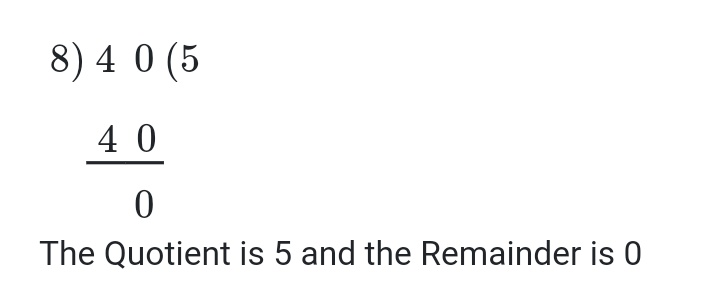Explanation:

Since you subtract 5 times
There are 5 groups of 8 in 40
40 divided by 8 equals 5

Question 8.
9)$$\bar{ 3 6 }$$
________Explanation:

You subtract 36 and 9 by 4 times
There are 4 groups of 9 in 36
So, 36 divided by 9 equals 4

Question 9.
Mrs. Costa has 18 pencils. She gives 9 pencils to each of her children for school. How many children does Mrs. Costa have?
________ children

Explanation:

Given,
Mrs. Costa has 18 pencils
She gives 9 pencils to each of her children for school
Divide total number of pencils by no. pencils to each of her children for school
18 ÷ 9 = 2 pencils

Question 10.
Boël decides to plant rose bushes in her garden. She has 24 bushes. She places 6 bushes in each row. How many rows of rose bushes does she plant in her garden?
________ rows

Explanation:

Boel has 24 bushes
she places 6 bushes in each row
To know the number of rows of rose bushes does she plant in her garden
We have to divide the total number of bushes by bushes in each row
= 24 ÷ 6 = 24/6 = 4

### Relate Subtraction and Division Page No 330

Question 1.
Which division equation is shown?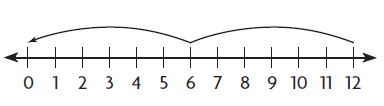Options:
a. 3 × 4 = 12
b. 12 ÷ 6 = 2
c. 12 ÷ 3 = 4
d. 12 ÷ 4 = 3

Answer: 12 ÷ 6 = 2

Explanation:

Step 1:

Count starts at 12

Step 2:

Count back by 6s as many times as you can until you get 0

Step 3:

Count the number of times you jumped back
You jumped back 2 times
There are 2 jumps from 12 to 0
So the correct answer is option B

Question 2.
Isabella has 35 cups of dog food. She feeds her dogs 5 cups of food each day. For how many days will the dog food last?
Options:
a. 6 days
b. 7 days
c. 8 days
d. 9 days

Explanation:

Given that,

Isabella has 35 cups of dog food
She feeds her dogs 5 cups of food each day
To find the number of days will the dog food last
We have to divide number of cups by the number of cups of food each day
= 35 ÷ 5 = 35/5 = 7
So, the correct answer is option B

Spiral Review

Question 3.
Ellen buys 4 bags of oranges. There are 6 oranges in each bag. How many oranges does Ellen buy?
Options:
a. 10
b. 12
c. 24
d. 30

Explanation:

Given:
Ellen buys 4 bags of oranges
There are 6 oranges in each bag
Total number of oranges = no. of bags × no. of oranges in each bag
= 4 × 6 = 24
Therefore the correct answer is option B

Question 4.
Each month for 7 months, Samuel mows 3 lawns. How many more lawns does he need to mow before he has mowed 29 lawns?
Options:
a. 1
b. 3
c. 7
d. 8

Explanation:

Each month for 7 months, Samuel mows 3 lawns
For one month Samuel mows 3 lawns
For 7 months Samuel mows = x
x = 7 × 3 = 21
Now, we need to know how many more lawns does he need to mow before he has mowed 29 lawns
Subtract 21 from 29
= 29 – 21 = 8
So the answer is option D

Use the graph for 5–6.Question 5.
How many hours did Eli volunteer?
Options:
a. 4 hours
b. 8 hours
c. 9 hours
d. 10 hours

Explanation:

The bar graph shows that Eli volunteered 9 hours
So, the correct answer is option C

Go Math Grade 3 Chapter 6 Review/Test Pdf Question 6.
Madi volunteered 2 hours less than Jill. At what number should the bar for Madi end?
Options:
a. 3
b. 6
c. 8
d. 12

Explanation:

The figure shows that Jill has volunteered 10 hours
If Madi has volunteered 2 hours less than Jill
= 10 – 2 = 8 hours
Thus the correct answer is option C

### Mid-Chapter Checkpoint Page No 331

Vocabulary

Choose the best term from the box to complete the sentence.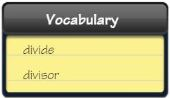Question 1.
You _________ when you separate into equal groups.
_________

When you divide, you separate into equal groups.

Concepts and Skills

Use counters or draw a quick picture on your MathBoard.
Make or circle equal groups. Complete the table.

Question 2.Type below:
___________

2. Number of counters = 6
Number of equal groups = 2
Number in each group =  __
= 6/2 = 3

3. Number of counters = 30
Number of equal groups = __
Number in each group = 5
= 30/5 = 6

4. Number of counters = 28
Number of equal groups = 7
Number in each group = __
= 28/7 = 4

Write a division equation for the picture.

Question 5.Type below:
___________

Answer: 8 ÷ 4 = 2 or 8 ÷ 2 = 4

Explanation:

The number of counters is 8 and a circle group of 4 counters.
Continue circling group of 4 until all the 8 counters are in the group
Divide Number of counters by Number of equal groups
= 8 ÷ 2 = 4
Divide Number of counters by number in each group
8 ÷ 4 = 2

Question 6.Type below:
___________

Answer: 15 ÷ 3 = 5 or 15 ÷ 5 = 3

Explanation:

Number of counters = 15
Number of groups = 3
Number in each group = 5
Divide Number of counters by number of groups
= 15 ÷ 3 = 5
Divide number of counters by number in each group
= 15 ÷ 5 = 3

Write a division equation.

Question 7.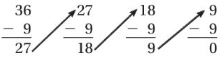______ ÷ ______ = ______

Answer: 36 ÷ 9 = 4

Explanation:

Step 1:

Starts at 36

Step 2:

Subtract with 9 until you get 0

Step 3:

Count the number of times you subtract with 9

You subtract 4 times
There are 4 groups of 9 with 36
So, 36 ÷ 9 = 4

Question 8.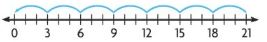______ ÷ ______ = ______

Answer: 21 ÷ 3 = 7

Explanation:

Step 1:

Starts at 21

Step 2:

Count back by 3s as many times as you can

Step 3:

Count the number of times you jumped back 3.
You jumped back by 21 seven times
There are 7 jumps of 3 in 21

### Mid-Chapter Checkpoint Page No 332

Question 9.
Victor plants 14 seeds in some flowerpots. If he puts 2 seeds in each pot, how many flowerpots does he use?
_________ flowerpots

Explanation:

Victor plants 14 seeds in some flowerpots
If he puts 2 seeds in each pot
Number of flowerpots he uses = x
x = 14/2
x = 7
So, Victor used 7 flowerpots

Question 10.
Desiree had 35 stickers. She gave each of 3 friends the same number of stickers. She now has 20 stickers left. She then gives the same number of stickers to each of another 5 friends. How many stickers did she give each of her 3 friends? Each of her 5 friends?
Type below:
___________

Answer: 5 stickers for 3 friends; 4 stickers for 5 friends

Explanation:

Given,
She gave each of 3 friends the same number of stickers
She now has 20 stickers left
To know how many stickers she gave to her friends
We have to subtract the number of stickers left from total number of stickers
35 – 20 = 15
That means she gave 15 stickers to her 3 friends equally
15 ÷ 3 = 5 stickers
So, each friend gets 5 stickers
Next, she gave remaining 20 stickers to 5 friends equally
20 ÷ 5 = 4
So, each friend gets 4 stickers

Question 11.
Jayden modeled a division equation with some counters. What division equation could Jayden have modeled?Type below:
___________

Answer: 14 ÷ 2 = 7 or 14 ÷ 7 = 2

Explanation:

Number of counter = 14
Number in each group = 7
Number of equal groups = 2
So, the division equation is the number of counters by the number of equal groups = 14 ÷ 2 = 7
Or, the Number of counters by number in each group = 14 ÷ 7 = 2

Go Math Grade 3 Chapter 6 Test Pdf Question 12.
Lillian bought 24 cans of cat food. There were 4 cans in each pack. How many packs of cat food did Lillian buy?_________ packs

Explanation:

Lillian bought 24 cans of cat food
There were 4 cans in each pack
Number of packs of cat food did Lillian buy
24 ÷ 4 = 6 packs

### Model with Arrays Page No 337

Use square tiles to make an array. Solve

Question 1.
How many rows of 4 are in 12?
______ rows

■ ■ ■ ■
■ ■ ■ ■
■ ■ ■ ■

Explanation:

Step 1:

Total number of tiles are 12

Step 2:
Make a row of 4 tiles

Step 3:

Continue to make as many rows of 4 tiles as you can
We get 4 tiles in each row
3 rows of 4 are in 12

Question 2.
How many rows of 3 are in 21?
______ rows

■ ■ ■
■ ■ ■
■ ■ ■
■ ■ ■
■ ■ ■
■ ■ ■
■ ■ ■

Explanation:

Step 1:

Total number of tiles are 21

Step 2:

Make a row of 3 tiles

Step 3:

Continue to make as many rows of 3 tiles as you can
We get 3 tiles in each row
So, 7 rows of 3 are in 21

Question 3.
How many rows of 6 are in 30?
______ rows

■ ■ ■ ■ ■ ■
■ ■ ■ ■ ■ ■
■ ■ ■ ■ ■ ■
■ ■ ■ ■ ■ ■
■ ■ ■ ■ ■ ■

Explanation:

Step 1:

First of all the count number of tiles = 30

Step 2:

Make a row of 6 tiles

Step 3:

Continue to make as many rows of 6 tiles as you can
We get 6 tiles in each row
So, there are 5 rows of 6 in 30

Question 4.
How many rows of 9 are in 18?
______ rows

Make an array. Then write a division equation.

Question 5.
20 tiles in 5 rows
______ ÷ ______ = ______

Answer: 20 ÷ 5 = 4

■ ■ ■ ■
■ ■ ■ ■
■ ■ ■ ■
■ ■ ■ ■
■ ■ ■ ■

Explanation:

Total number of tiles = 20
Make a row of 4 tiles
Continue to make as many rows of 4 tiles as you can
We get 4 tiles in each row
So, the division equation is 20 ÷ 5 = 4

Question 6.
28 tiles in 7 rows
______ ÷ ______ = ______

Answer: 28 ÷ 7 = 4

■ ■ ■ ■
■ ■ ■ ■
■ ■ ■ ■
■ ■ ■ ■
■ ■ ■ ■
■ ■ ■ ■
■ ■ ■ ■

Explanation:

Total number of tiles = 28
Make a row of 4 tiles
Continue to make as many rows of 4 tiles as you can
We get 4 tiles in each row
For 28 tiles we get 7 rows
So, the division equation is 28 ÷ 7 = 4

Question 7.
18 tiles in 9 rows
______ ÷ ______ = ______

Answer: 18 ÷ 9 = 2

■ ■
■ ■
■ ■
■ ■
■ ■
■ ■
■ ■
■ ■
■ ■

Explanation:

Number of tiles = 18
Number of rows = 9
Now we have to make a row of 2 tiles until we complete 18 tiles
So, you get 2 tiles in 9 rows
18 ÷ 9 = 2 tiles

Question 8.
36 tiles in 6 rows
______ ÷ ______ = ______

Answer: 36 ÷ 6 = 6

■ ■ ■ ■ ■ ■
■ ■ ■ ■ ■ ■
■ ■ ■ ■ ■ ■
■ ■ ■ ■ ■ ■
■ ■ ■ ■ ■ ■
■ ■ ■ ■ ■ ■

Explanation:

Total number of tiles = 36
Number of rows = 6
Now you have to make a row of 36 tiles in 6 rows
You get 6 tiles in each row
That means there are 6 tiles each in a row
So, the division equation is 36 ÷ 6 = 6

Problem Solving

Question 9.
A dressmaker has 24 buttons. He needs 3 buttons to make one dress. How many dresses can he make with 24 buttons?
______ dresses

Explanation:

Given: A dressmaker has 24 buttons
He needs 3 buttons to make one dress
Number of dresses he can make with 24 buttons = y
y × 3 = 24
y = 24/3
y = 8

Go Math 3rd Grade Chapter 6 Review Test Question 10.
Liana buys 36 party favors for her 9 guests. She gives an equal number of favors to each guest. How many party favors does each guest get?
______ party favors

Explanation:

Liana buys 36 party favors for her 9 guests.
She gives an equal number of favors to each guest.
To know how many party favors a guest get
We must Divide the number of 36 party favors by 9 guests
= 36 ÷ 9 = 4

### Model with Arrays Page No 338

Question 1.
Mr. Canton placed 24 desks in 6 equal rows. How many desks are in each row?
Options:
a. 2
b. 3
c. 4
d. 5

Explanation:

Mr. Canton places 24 desks in 6 equal rows
Each row has x number of desks
Divide the number of desks by number of equal rows
24 ÷ 6 = 4
So, the correct answer is option C

Question 2.
Which division equation is shown by the array?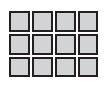Options:
a. 12 ÷ 6 = 2
b. 12 ÷ 3 = 4
c. 12 ÷ 2 = 6
d. 12 ÷ 1 = 12

Answer: 12 ÷ 3 = 4

Explanation:

The number of counters is 12 and the number of rows is 3
To know number counters in each row
We have to divide the number of counters by the number of rows
= 12 ÷ 3 = 4
Thus the answer is option B

Spiral Review

Question 3.
Amy has 2 rows of 4 sports trophies on each of her 3 shelves. How many sports trophies does Amy have in all?
Options:
a. 8
b. 9
c. 12
d. 24

Explanation:

Amy has 2 rows of 4 sports trophies on each of her 3 shelves
To know the total sports trophies does Amy have in all 3 shelves
We have to multiple number 2 × 4 × 3 = 24
So, the correct answer is option D

Question 4.
What is the unknown factor?
9 × p = 45
Options:
a. 4
b. 5
c. 6
d. 7

Explanation:

The unknown factor is p
9 × p = 45
p = 45/9 = 5
Therefore p = 5
Thus the answer is option B

Question 5.
Sam has 7 stacks with 4 quarters each. How many quarters does Sam have?
Options:
a. 11
b. 12
c. 24
d. 28

Explanation:

Sam has 7 stacks with 4 quarters each
Each stack has 4 quarters
So, 7 stacks has 7 × 4 = 28
Thus 7 stacks have 28 quarters

Question 6.
How can you skip count to find how many counters in all?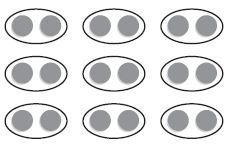Options:
a. 3 groups of 2
b. 3 groups of 3
c. 9 groups of 2
d. 18 groups of 2

Explanation:

Total number of Counters = 18
Number of equal groups = 9
Number in each group = 2
So, there are 9 groups of 2s
Thus the correct answer is option C

### Relate Multiplication and Division Page No 343

Complete the equations.

Question 1.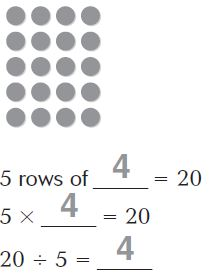5 rows of 4 = 20
5 × 4  = 20
20 ÷ 5 = 4

Explanation:

The above figure shows that, there are 5 rows of 4 circles
Total number of circles = 20
The related facts of 20, 5 and 4 are
5 × 4 = 20; 5 rows of 4 = 20; 20 ÷ 5 = 4

Question 2.4 rows of ______ = 24
4 × ______ = 24
24 ÷ 4 = ______

4 rows of 6 = 24
4 × 6 = 24
24 ÷ 4 = 6

Explanation:

Number of counters = 24
Number of equal rows = 4
24 ÷ 4 = 6
The related facts of 24, 6 and 4 are 24 ÷ 4 = 6; 4 × 6 = 24

Question 3.3 rows of ______ = 24
3 × ______ = 24
24 ÷ 3 = ______

3 rows of 8 = 24
3 × 8 = 24
24 ÷ 3 = 8

Explanation:

Total number of counters = 24
Number of equal rows = 3
Divide number of counters by number of equal rows
24 ÷ 3 = 8
Thus the related multiplication and division facts of 24, 3 , 8 are 3 × 8 = 24; 24 ÷ 3 = 8

Complete the equations.

Question 4.
4 × ______ = 28   28 ÷ 4 = ______

Explanation:
The related facts of 28 and 4 are 28 ÷ 4 = 7 and 4 × 7 = 28

Question 5.
6 × ______ = 36    36 ÷ 6 = ______

Explanation:

Let the unknown Number be x
6 × x = 36
x = 36/6 = 6
The related multiplication and division facts of 36 and 6 are 36 ÷ 6 = 6; 6 × 6 = 36

Question 6.
5 × ______ = 35    35 ÷ 7 = ______

Explanation:

X represents the unknown factor
5 × x = 35
x = 35/5 = 7
Therefore the related multiplication and division facts 35, 5 and 7 are 5 × 7 = 35; 35 ÷ 7 = 5

Question 7.
7 × ______ = 21  21 ÷ 7 = ______

Explanation:

7 × x = 21
x = 21/7 = 3
So, the related multiplication and division facts of 7, 21 are 7 × 3 = 21; 21÷ 7 = 3

Question 8.
9 × ______ = 27  27 ÷ 9 = ______

Explanation:

27 ÷ 9 = 3
The related multiplication and division facts of 27 and 9 are 9 × 3 = 27 and 27 ÷ 9 = 3

Question 9.
2 × ______ = 16  16 ÷ 2 = ______

Explanation:

16 ÷ 2 = 8
The related multiplication and division facts of 16 and 2 are 2 × 8 = 16; 16 ÷ 2 = 8

Question 10.
4 × ______ = 36 36 ÷ 4 = ______

Explanation:

36 ÷ 4 = 9
So, the related multiplication and division facts of 36 and 4 are 4 × 9 = 36; 36 ÷ 4 = 9

Question 11.
8 × ______ = 40 40 ÷ 8 = ______

Explanation:

Let x be the unknown factor
8 × x = 40
x = 40/8 = 5
The related facts of 40 and 8 are 8 × 5 = 40; 40 ÷ 8 = 5

Problem Solving

Question 12.
Mr. Martin buys 36 muffins for a class breakfast. He places them on plates for his students. If he places 9 muffins on each plate, how many plates does Mr. Martin use?
___________ plates

Explanation:

Number of muffins = 36
He places 9 muffins on each plate
Divide number of muffins by number of muffins on each plate
36 ÷ 9 = 4 plates

Question 13.
Ralph read 18 books during his summer vacation. He read the same number of books each month for 3 months. How many books did he read each month?
___________ books

Explanation:

Given that Ralph read 18 books during his Summer vacation
He read the same number of books each month for 3 months
To find the number of books he read each month we have to divide the total number of books by the number of books for 3 months
18 ÷ 3 = 6 books

### Relate Multiplication and Division Page No 344

Question 1.
Which number will complete the equations?
6 × □ = 24
24 ÷ 6 = □
Options:
a. 3
b. 4
c. 5
d. 6

Explanation:

24 ÷ 6 = 4
The related multiplication and division facts of 6 and 24 is 6 × 4 = 24; 24 ÷ 6 = 4
So, the correct answer is option B

Question 2.
Alice has 14 seashells. She divides them equally between her 2 sisters. How many seashells does each sister get?
Options:
a. 7
b. 8
c. 12
d. 16

Explanation:

Alice has 14 seashells
She divides them equally between her 2 sisters
To know the number of seashells each sister get
We have to divide number of seashells by the number of sisters
= 14 ÷ 2 = 7
Thus the answer is option A

Spiral Review

Question 3.
Sam and Jesse can each wash 5 cars in an hour. They both work for 7 hours over 2 days. How many cars did Sam and Jesse wash?
Options:
a. 70
b. 35
c. 24
d. 14

Explanation:

Sam and Jesse can each wash 5 cars in an hour
They both work for 7 hours over 2 days
To find the total number of cars they washed
we need to multiply 5 × 7 × 2 = 35 × 2 = 70
So, the answer is option A

Question 4.
Keisha skip-counted to find how many counters in all. How many equal groups are there?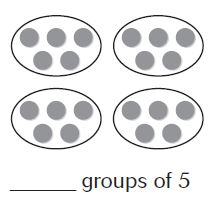Options:
a. 3
b. 4
c. 5
d. 20

Explanation:

By seeing the above figure we can say that there are 4 groups.
So, the answer is option B

Question 5.
The key for a picture graph showing the number of books students read is: Each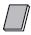= 2 books. How many books did Nancy read if she has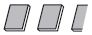by her name?
Options:
a. 2
b. 4
c. 5
d. 6

Explanation:

Each= 2 books
There are 2 and half books = 2 + 2 + 1 = 5
Thus the answer is 5 i.e., option C

Question 6.
Jan surveyed her friends to find their favorite season. She recorded IIII III for summer. How many people chose summer as their favorite season?
Options:
a. 5
b. 8
c. 9
d. 13

Explanation:

IIII = 5
III = 3
IIII III = 5 + 3 = 8
So, the people who chose summer as their favorite season are 8
Option B is the correct answer

### Write Related Facts Page No 349

Write the related facts for the array.

Question 1.2 × 6 = 12
6 × 2 = 12
12 ÷ 2 = 6
12 ÷ 6 = 2

Explanation:

Total number of counters = 12
Number of rows = 2
Number of counters in each row = 6
So, the related facts of 6, 2 and 12 are 2 × 6 = 12, 6 × 2 = 12, 12 ÷ 2 = 6 and 12 ÷ 6 = 2

Question 2.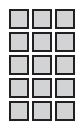Type below:
_________

5× 3 = 15
3×5 = 15
15 ÷ 3 = 5
15÷ 5 = 3

Explanation:

Total number of counters = 15
Number of rows = 5
Number of counters in each row = 3
The related facts of 5, 3 and 15 are 5× 3 = 15, 3×5 = 15, 15 ÷ 3 = 5 and 15÷ 5 = 3

Question 3.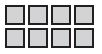Type below:
_________

2 × 4 = 8
4× 2 = 8
8 ÷ 2 = 4
8 ÷ 4 = 2

Explanation:

Total number of counters = 8
Number of rows = 2
Number of counters in each row = 4
The related facts of 8, 2, 4 are 2 × 4 = 8, 4× 2 = 8, 8 ÷ 2 = 4 and 8 ÷ 4 = 2

Write the related facts for the set of numbers.

Question 4.
3, 7, 21
Type below:
_________

3 × 7 = 21
7 × 3 = 21
21 ÷ 3 = 7
21 ÷ 7 = 3

Explanation:

Total number of counters = 21
The related facts of 3, 7, 21 are 3 × 7 = 21, 7 × 3 = 21, 21 ÷ 3 = 7 and 21 ÷ 7 = 3

Question 5.
2, 9, 18
Type below:
_________

2 × 9 = 18
9 × 2 = 18
18 ÷ 2 = 9
18 ÷ 9 = 2

Explanation:

Total number of counters = 18
The related facts of 2, 9, 18 are 2 × 9 = 18, 9 × 2 = 18, 18 ÷ 2 = 9 and 18 ÷ 9 = 2

Question 6.
4, 8, 32
Type below:
_________

4 × 8 = 32
8 × 4 = 32
32 ÷ 8 = 4
32 ÷ 4 = 8

Explanation:

Total number of counters = 32
The related facts of 4, 8, 32 are 4 × 8 = 32, 8 × 4 = 32, 32 ÷ 8 = 4 and 32 ÷ 4 = 8

Complete the related facts.

Question 7.
4 × 9 = _______
9 × _______ = 36
36 ÷ _______ = 4
_______ ÷ 4 = 9

Answer: The related facts of 4, 9 and 36 are given below

4 × 9 = 36
9 × 4 = 36
36 ÷ 9 = 4
36 ÷ 4 = 9

Question 8.
_______ × 7 = 35
_______ × 5 = 35
_______ ÷ 7 = 5
35 ÷ 5 _______

5 × 7 = 35
7 × 5 = 35
35 ÷ 7 = 5
35 ÷ 5= 7

Explanation:

Let the unknown factor be x
x × 7 = 35
x = 35/7 = 5
5 × 7 = 35

x × 5 = 35
x = 35/5 = 7
7 × 5 = 35

x ÷ 7 = 5
x = 5 × 7 = 35
35 ÷ 7 = 5

35 ÷ 5 = x
x = 35/5 = 7
35 ÷ 5= 7

Question 9.
6 × _______ = 18
3 × 6 _______
18 ÷ _______ = 3
_______ ÷ 3 = 6

6 × 3 = 18
3 × 6 = 18
18 ÷ 6 = 3
18 ÷ 3 = 6

Explanation:

Let the unknown factor be x
6 × x = 18
x = 18/6 = 3
6 × 3 = 18

3 × 6 = x
x = 18
3 × 6 = 18

18 ÷ x = 3
x = 18/3 = 6
18 ÷ 6 = 3

x ÷ 3 = 6
x = 6 × 3 = 18
18 ÷ 3 = 6

Problem Solving

Question 10.
CDs are on sale for $5 each. Jennifer has$45 and wants to buy as many as she can. How many CDs can Jennifer buy?
_______

Explanation:

CDs are on sale for $5 each Jennifer has$45 and wants to buy as many as she can
= 45 ÷ 5 = 9
so, the number of CDs can Jennifer buy = 9

Grade 3 Chapter 6 Understand Multiplication and Division Question 11.
Mr. Moore has 21 feet of wallpaper. He cuts it into sections that are each 3 feet long. How many sections does Mr. Moore have?
_______ sections

Explanation:

Mr. Moore has 21 feet of wallpaper
He cuts it into sections that are each 3 feet long
Number of sections does Mr. Moore have = x
= 21/3 = 7
Mr. Moore has 7 sections

### Write Related Facts Page No 350

Question 1.
Which number completes the set of related facts?
5 × □ = 40  40 ÷ □ = 5
□ × 5 = 40  40 ÷ 5 = □
Options:
a. 6
b. 7
c. 8
d. 9

Explanation:

i. 5 × □ = 40
□ = 40/5 = 8
ii. 40 ÷ □ = 5
□ = 40/5 = 8
iii. □ × 5 = 40
□ = 40/5 = 8
iv. 40 ÷ 5 = □
40/5 = □
□ = 8

Question 2.
Which equation is not in the same set of related facts as 4 × 7 = 28?
Options:
a. 7 × 4 = 28
b. 4 + 7 = 11
c. 28 ÷ 4 = 7
d. 28 ÷ 7 = 4

Answer: 4 + 7 = 11

Explanation:

The related facts of 4, 7 and 28 are 4 × 7 = 28, 7 × 4 = 28, 28 ÷ 4 = 7, 28 ÷ 7 = 4
But 4 + 7 = 11 is not set of related multiplication and division equations.

Spiral Review

Question 3.
Beth runs 20 miles each week for 8 weeks. How many miles does Beth run in 8 weeks?
Options:
a. 16 miles
b. 28 miles
c. 100 miles
d. 160 miles

Explanation:

Beth runs 20 miles each week for 8 weeks
Each week Beth runs 20 miles
For 8 weeks = x miles
x = 8 × 20
x = 160 miles
So, the answer is 160 miles

Question 4.
Find the product.
5 × 0
Options:
a. 0
b. 1
c. 5
d. 10

Explanation:

Any number multiplied with 0 is always 0.
So, the answer is option A.

Question 5.
Uri’s bookcase has 5 shelves. There are 9 books on each shelf. How many books in all are in Uri’s bookcase?
Options:
a. 14
b. 36
c. 45
d. 54

Explanation:

Uri’s bookcase has 5 shelves
There are 9 books on each shelf
Number of books in 5 shelves = y
y = 9 × 5 = 45
Thus the answer is option C

Question 6.
There are 6 batteries in one package. How many batteries will 6 packages have?
Options:
a. 12
b. 18
c. 24
d. 36

Explanation:

There are 6 batteries in one package
Number of batteries in 6 packages = x
x = 6 × 6 = 36
Thus the Number of batteries in 6 packages = 36

### Division Rules for 1 and 0 Page No 355

Find the quotient.

Question 1.Explanation:

Any number divided by 1 equals that number. The quotient is that number
So, 3/1 = 3 is the quotient

Question 2.
8 ÷ 8 = ________

Explanation:

Any number divided by the same number other than 0 equals 1. The quotient is always 1
8/8 = 1
So, the quotient is 1

Question 3.
________ = 0 ÷ 6

Explanation:

Zero divided by any number is always 0. The quotient is 0.
0/6 = 0
Thus the quotient is 0

Question 4.
2 ÷ 2 = ________

Explanation:

Any number divided by the same number equals 1. The quotient is always 1
2/2 = 1
Thus the quotient is 1

Question 5.
________ = 9 ÷ 1

Explanation:

Any number divided by 1 equals that number. The quotient is that number
9/1 = 9
So, the quotient is 9

Question 6.
0 ÷ 2 = ________

0 divided by any number is always 0.
0/2 = 0
So, the quotient is 0

Question 7.
0 ÷ 3 = ________

Explanation:

0 divided by any number is always 0.
0/3 = 0
So, the quotient is 0

Question 8.
________ = 0 ÷ 4

Explanation:

0 divided by any number is 0
0/4 = 0
Thus the quotient is 0

Question 9.
7)$$\bar{ 7 }$$
________

Explanation:

Any number divided by the same number is always 1.
7/7 = 1
So, the quotient is 1

Question 10.
1)$$\bar{ 6 }$$
________

Explanation:

Any number divided by 1 is the same number.
6/1 = 6
So, the quotient is 6

Question 11.
9)$$\bar{ 0 }$$
________

Explanation:

0 divided by any number remains 0.
0/9 = 0
Thus the quotient is 0

Question 12.
1)$$\bar{ 5 }$$
________

Explanation:

Any number divided by 1 gives the same number as a quotient
5/1 = 5
Therefore the quotient is 5

Question 13.
1)$$\bar{ 0 }$$
________

Explanation:

0 divided by any thing is 0
0/1 = 0
So, the quotient is 0

Question 14.
4)$$\bar{ 4 }$$
________

Explanation:

Any number divided by the same number gives the quotient as 1.
4/4 = 1
So, the quotient is 1

Question 15.
1)$$\bar{ 10 }$$
________

The number which is divided by 1 gives the same number as a quotient.
10/1 = 10
Thus the quotient is 10

Question 16.
2)$$\bar{ 2 }$$
________

Explanation:

Any number divided by the same number is 1.
2/2 = 1
Thus the quotient is 1

Problem Solving

Question 17.
There are no horses in the stables. There are 3 stables in all. How many horses are in each stable?
________

Explanation:

If there are no horses then there will be nothing in the stables.
Zero divided by any number is 0
0/3 = 0

Question 18.
Jon has 6 kites. He and his friends will each fly 1 kite. How many people in all will fly a kite?
________

Explanation:

John has 6 kites
He and his friends will each fly 1 kite
= 6/1 = 6
So, 6 people will fly the kites

### Division Rules for 1 and 0 Page No 356

Question 1.
Candace has 6 pairs of jeans. She places each pair on its own hanger. How many hangers does Candace use?
Options:
a. 0
b. 1
c. 6
d. 12

Explanation:

Given,
Candace has 6 pairs of jeans
She places each pair on its own hanger
That means one pair of jeans for one hanger
To find the number of hangers we need to divide the total number of hangers by each pair
6 ÷ 1 = 6
So, option C is the correct answer

Question 2.
There are 0 birds and 4 bird cages. Which division equation describes how many birds are in each cage?
Options:
a. 0 ÷ 4 = 0
b. 4 ÷ 4 = 1
c. 4 ÷ 1 = 4
d. 0 × 4 = 0

Answer: 0 ÷ 4 = 0

Explanation:

There are no birds so nothing to divide. Zero divided by anything is 0.
So, the answer is 0 ÷ 4 = 0

Spiral Review

Question 3.
There are 7 plates on the table. There are 0 sandwiches on each plate. How many sandwiches are on the plates in all?
7 × 0
Options:
a. 0
b. 1
c. 7
d. 70

Explanation:

There are 7 plates on the table
There are 0 sandwiches on each plate
Any number multiplied with 0 is always 0.
So, the answer is option A.

Question 4.
Which shows a way to break apart the array to find the product?Options:
a. (3 × 5) + (3 × 2)
b. (2 × 8) + (1 × 8)
c. (4 × 7) + (1 × 7)
d. (3 × 6) + (3 × 3)

Answer: (3 × 5) + (3 × 2)

Explanation:

There are 3 rows and 7 columns
The columns are divided into 2 parts 5 and 2.
By using the distributive property we can write it as (3 × 5) + (3 × 2)
Thus the answer is option A

Question 5.
Which of the following describes a pattern in the table?Options:
b. Multiply by 2.
c. Subtract 1.
d. Multiply by 6.

Multiply by 6.

Explanation:

Multiple 6 with a number of vans.
By seeing the above table we can say that it is the multiple of 6.
So, the answer is option D

Question 6.
Use the graph.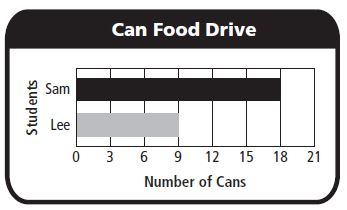How many more cans did Sam bring in than Lee?
Options:
a. 4
b. 5
c. 7
d. 9

Explanation:

Number of cans sam brings = 18
Number of cans Lee bring = 9
To know number of cans Sam bring in than Lee we have to subtract
Number of cans of Lee from Number of cans of Sam = 18 – 9 = 9
By using the above graph we can say that number of cans Sam bring in than Lee is 9.
Thus the correct answer is option D

### Review/Test Page No 361

Question 1.
For numbers 1a–1d, select True or False for each equation.
a. 3 ÷ 1 = 1
i. True
ii. False

Explanation:

Any number divided by 1 is always the same number. The quotient is 1.
So, the above equation is false.

Question 1.
b. 0 ÷ 4 = 0
i. True
ii. False

Explanation:

0 divided by any number is always 0. So, the above statement is true.

Question 1.
c. 7 ÷ 7 = 1
i. True
ii. False

Explanation:

Any number divided by the same number remains 1. So, the given statement is true.

Question 1.
d. 6 ÷ 1 = 6
i. True
ii. False

Explanation:

Any number divided by 1 will be the same number. Thus the statement given above is true.

Question 2.
Elizabeth has 12 horses on her farm. She puts an equal number of horses in each of 3 pens. How many horses are in each pen?
Circle a number that makes the sentence true.
There are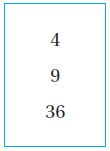horses in each pen.
_________

Answer: 12 ÷ 3 = 4

There are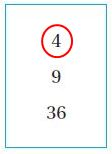horses in each pan

Question 3.
Chris plants 25 pumpkins seeds in 5 equal rows. How many seeds does Chris plant in each row?
Make an array to represent the problem. Then solve the problem.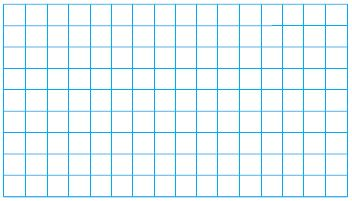_______ seeds
Explain:
_________Explanation:

Total number of seeds = 25
Number of equal rows = 5
25 ÷ 5 = 5 seeds

Page No. 358

Question 4.
Becca spent 24 minutes walking around a track. It took her 3 minutes to walk each time around the track. How many times did Becca walk around the track?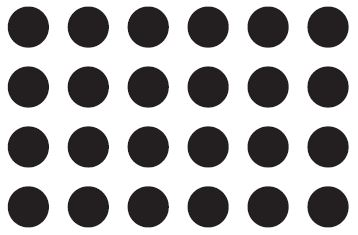Make equal groups to model the problem. Then explain how you solved the problem.
_______ times

Answer: 24 ÷ 3 = 8 timesQuestion 5.
There are 7 cars in an amusement park ride. There are 42 people divided equally among the 7 cars. An equal number of people ride in each car. How many people ride in one car?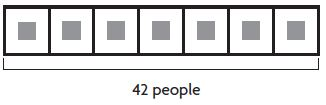_______people

Explanation:

Number of cars = 7
There are 42 people divided equally among the 7 cars
Divide the total number of people by the number of cars
You get, 42 ÷ 7 = 6 people

Question 6.
Select the equations that represent the array. Mark all that apply.Options:
a. 3 × 5 = ■
b. 2 × ■ = 12
c. ■ ÷ 3 = 5
d. 5 × ■ = 15
e. 12 ÷ 3 = ■
f. 15 ÷ 5 = ■

Answer: 3 × 5 = ■; ■ ÷ 3 = 5; 5 × ■ = 15; 15 ÷ 5 = ■

Explanation:

Related facts are a set of related multiplication and division equations.

Number of rows = 3
Number of blocks in each row = 5
Total Number of blocks = 15
So, the relation between these 3 numbers are 3 × 5 = ■; ■ ÷ 3 = 5; 5 × ■ = 15; 15 ÷ 5 = ■

### Review/Test Page No 359

Question 7.
Eduardo visited his cousin for 28 days over the summer. There are 7 days in each week. How long, in weeks, was Eduardo’s visit?
Part A
Draw jumps on the number line to model the problem.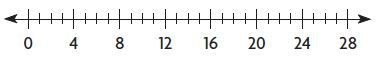Type below:
___________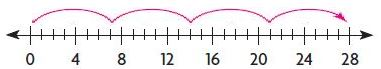Starts at 0
Count by 7 as many times as you can
Count the number of times you jumped back 7
You have jumped 4 times
So, there are 4 groups of 7 in 28
28 ÷ 7 = 4

Question 7.
Part B
Write a division equation to represent the model.
Type below:
___________

Answer: 28 ÷ 7 = 4

Question 8.
A workbook is 64 pages long. If each chapter is 8 pages long, how many chapters are there?
_________ chapters

Explanation:

A workbook is 64 pages long
If each chapter is 8 pages long
Divide the number of pages by number of pages in each chapter
= 64 ÷ 8 = 64/8 = 8
Therefore there are 8 chapters in the workbook

Question 9.
There are 56 apples packed in 7 baskets with the same number of apples in each basket. How many apples are in each basket?
For numbers, 9a–9d, choose Yes or No to tell whether the equation represents the problem.
a. 56 + 7 = ■
i. yes
ii. no

Explanation:

56 + 7 = 63 ≠ 8

Question 9.
b. 7 × ■ = 56
i. yes
ii. no

Explanation:

7 × ■ = 56
■ = 56/7 = 8

Question 9.
c. 56 ÷ ■ = 8
i. yes
ii. no

Explanation:

56 ÷ ■ = 8
■ = 56/8 = 7

Question 9.
d. 56 − ■ = 8
i. yes
ii. no

Explanation:

56 − ■ = 8
■ = 64 ≠ 7

Question 10.
Stefan has 24 photos to display on some posters. Select a way that he could display the photos in equal groups on the posters. Mark all that apply.
Options:
a. 6 photos on each of 4 posters
b. 7 photos on each of 3 posters
c. 4 photos on each of 6 posters
d. 5 photos on each of 5 posters
e. 3 photos on each of 8 posters
f. 7 photos on each of 4 posters

A. 6 photos on each of 4 posters
6 x 4 = 24 photos ✓

B. 7 photos on each of 3 posters
7 x 3 = 21 ≠ 24 photos

C. 4 photos on each of 6 posters
4 x 6 = 24 photos ✓

D. 5 photos on each of 5 posters
5 x 5 = 25 ≠ 24 photos

E. 3 photos on each of 8 posters
3 x 8 = 24 photos ✓

F. 7 photos on each of 4 posters
7 x 4 = 28 ≠ 24 photos

### Review/Test Page No 360

Question 11.
Debbie made this array to model a division equation. Which equation did Debbie model? Mark all that apply.Options:
a. 14 ÷ 7 = 2
b. 28 ÷ 4 = 7
c. 28 ÷ 7 = 4
d. 14 ÷ 2 = 7

Answer: 28 ÷ 4 = 7

Explanation:

Total Number shaded blocks = 28
Number of equal rows = 4
To write the division equation
we have to divide number of blocks by number of equal rows
28 ÷ 7 = 7
So, the correct answer is option B

Question 12.
Mrs. Edwards made a total of 40 fingers on some gloves she knitted. How many gloves did Mrs. Edwards knit?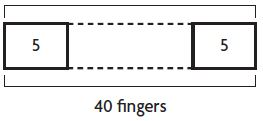__________ gloves

Answer: 40 ÷ 5 = 8

Explanation:

Mrs. Edwards made a total of 40 fingers on some gloves she knitted
Number in each group = 5
Divide Number of fingers by number in each group
= 40 ÷ 5 = 8
Number of equal groups = 8

Question 13.
Make true equations. Select a number to complete the equation.7 ÷ 7 = ________
7 ÷ 1 = ________
0 ÷ 7 = ________

7 ÷ 7 = 1
7 ÷ 1 = 7
0 ÷ 7 = 0

Explanation:

7 ÷ 7 = 1
Any number divided by the same numbers is always 1. So the quotient is 1
7 ÷ 1 = 7
Any number divided by 1 gives the same number as quotient.
0 ÷ 7 = 0
Zero divided by any number is always 0. So, the quotient is 0.

Question 14.
The coach separated the 18 players at lacrosse practice into 3 different groups. How many players were in each group?
________ players

Explanation:

Total number of players = 18
Number of equal groups = 3
To find the number in the equal group you have to divide the total number of players by number of equal groups
= 18 ÷ 3 = 6
Therefore there are 6 players in each group

### Review/Test Page No 361

Question 15.
Write a division equation to represent the repeated subtraction.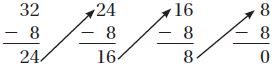Type below:
________

Answer: 32 ÷ 8 = 4

Explanation:

Step 1:

Start at 32

Step 2:

Subtract with 8 until you get 0

Step 3:

Count the number of times you subtract with 8
Since you subtract 4 times
There are 4 groups of 8 in 32
32 ÷ 8 = 4
Thirty two divided by eight equals four

Question 16.
Write related facts for the array. Explain why there are not more related facts.Type below:
________

There are 6 rows of 6 squares.
The related facts are:
6 x 6 = 36; 36 ÷ 6 = 6
An array represents a multiplication because it has rows and columns and the total number of cells is the product of its rows and columns.
Also, the division is related to multiplication.
So, the only related facts are multiplication and division.

Question 17.
Darius bakes 18 muffins for his friends. He gives each of his friends an equal number of muffins and has none left over.
Part A
Draw a picture to show how Darius divided the muffins and complete the sentence.
Darius gave muffins to ________ friends.
Type below:
________

If one muffin for 18 friends

18 = 1 × 18If 2 muffins for 9 friends

18 = 2 × 9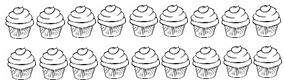If 3 muffins for 6 friends

18 = 3 × 6Six muffins for 3 friends

18 = 6 × 3

If 9 muffins for 2 friends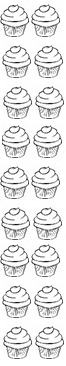So, Darius gave muffins to 2, 3, 6, 9, 18 friends

Question 17.
Part B
Could Darius have given all of his muffins equally to 4 of his friends? Explain why or why not.
Type below:
________

No, because if he divides 18 muffins to 4 people, then they get 4 muffins each, and two are not given.
4 × 4 + 2 = 18

### Review/Test Page No 362

Question 18.
Circle numbers to complete the related facts.× 8 = 72   72 ÷= 8
______                                              _______

Answer: 9 × 8 = 72; 72 ÷ 9 = 8× 8 = 72; 72 ÷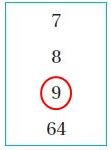= 8

Question 19.
Use the numbers to write a related multiplication and division facts.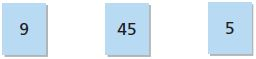Type below:
__________

Answer: 45 ÷ 9 = 5; 45 ÷ 5 = 9

Explanation:

The multiplication and division facts for 45, 9 and 5 are
45 ÷ 5 = 9
45 ÷ 9 = 5
9 × 5 = 45

Question 20.
Tyrone took 16 pennies from his bank and put them in 4 equal stacks. How many pennies did Tyrone put in each stack? Show your work.__________ pennies16 ÷ 4 = 4 pennies

Explanation:

Total number of pennies = 16
Number of equal stacks = 4
Divided number of pennies by number of equal stacks to know the pennies in each stack
16 ÷ 4 = 4

After solving the exercise and homework problems you can also try the questions provided at the end and middle of the chapter. Also, Check Go Math Grade 3 Answer Key Chapter 6 Understand Division Extra Practice to improve your math skills. This helps to know your knowledge over this chapter.

We have presented a detailed explanation of the problems to make you understand in an easy manner. Click on the links and start preparing for your exams. If you have any queries regarding Go Math Answer Key chapter 6 you can post your comments in the below comment box.

Scroll to Top